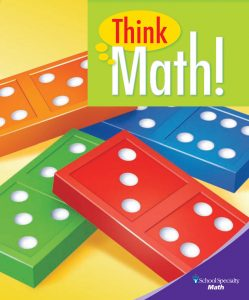## OverviewThink Math! is founded upon the principle that children are curious problem solvers. Each grade level’s content builds a puzzler’s disposition, developing mathematical “habits of mind” through games, puzzles, mental math, and other collaborative activities – with the goal of providing engaging and motivating experiences for a community of diverse learners.

In Think Math!, kindergarteners spend significant time representing whole numbers, beginning with numbers to 10 and eventually numbers to 20, using objects, pictures, drawings, and sounds. They use numbers to describe quantity and order and make comparisons using words such as “more than,” “greater than,” “less than,” “same as,” and “equal to” and are eventually introduced to >, <, and = symbols. Children eventually explore representing addition and subtraction as jumps on a number line, understanding that a “plus” jump moves forwards and a “minus” jump moves backwards. They sort and compare two-dimensional and three-dimensional shapes and describe common attributes.

All student materials can be accessed through the table below as PDFs by chapter.

## Chapter Listing

Chapter Topics Think Math! Resources
Chapter 1: Numbers to Ten
• Learning number names and digits
• Using numbers to describe quantities
• Using numbers to describe order
Chapter 1 Student Lesson Activity Book (LAB) pages

Chapter 1 Activity Masters Chapter 1 Flip Chart

Chapter 1 School-Home Connection Letter (English/Spanish)

Chapter 2: Two-Dimensional Figures and Quantities
• Identifying shape as an attribute
• Describing two-dimensional figures
• Recognizing figures in different orientations
Chapter 2 Student Lesson Activity Book (LAB) pages

Chapter 2 Activity Masters Chapter 2 Flip Chart

Chapter 2 School-Home Connection Letter (English/Spanish)

Chapter 3: Patterns and Sorting
• Sorting in more than one way
• Recording and sorting results
• Working with patterns
Chapter 3 Student Lesson Activity Book (LAB) pages

Chapter 3 Activity Masters Chapter 3 Flip Chart

Chapter 3 School-Home Connection Letter (English/Spanish)

Chapter 4: Moving on the Number Line
• Recognizing number patterns
• Combining jumps
Chapter 4 Student Lesson Activity Book (LAB) pages

Chapter 4 Activity Masters Chapter 4 Flip Chart

Chapter 4 School-Home Connection Letter (English/Spanish)

Chapter 5: Making and Breaking Numbers
• Joining and separating
• Decomposing and recomposing numbers
Chapter 5 Student Lesson Activity Book (LAB) pages

Chapter 5 Activity Masters Chapter 5 Flip Chart

Chapter 5 School-Home Connection Letter (English/Spanish)

Chapter 6: Stories in Numbers, Words, and Pictures
• Expressing a number as a sum in more than one way
• Separating wholes into two equal parts
Chapter 6 Student Lesson Activity Book (LAB) pages

Chapter 6 Activity Masters Chapter 6 Flip Chart

Chapter 6 School-Home Connection Letter (English/Spanish)

Chapter 7: Three-Dimensional Geometry
• Describing three-dimensional figures
• Describing the positions of objects
Chapter 7 Student Lesson Activity Book (LAB) pages

Chapter 7 Activity Masters

Chapter 7 School-Home Connection Letter (English/Spanish)

Chapter 8: Measurement
• Comparing and ordering objects
• Measuring objects
Chapter 8 Student Lesson Activity Book (LAB) pages

Chapter 8 Activity Masters Chapter 8 Flip Chart

Chapter 8 School-Home Connection Letter (English/Spanish)

Chapter 9: Large Numbers
• Working with larger numbers